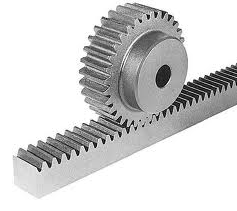## Motion Conversion (MEC214E)

This article shows some ways to convert motion in mechatronics applications. It is part of our book Mechatronics and Robotics Handbook (out of sale).

a) Rotary to longitudinal motion using a shaft and a piston

Figure 1 shows how a crank shaft and a piston can be used to convert rotary motion into longitudinal motion.

Formula

## D = 2 x R (f2.47)

Where:

D is the displacement of the piston

R is te length of the crankshaft

b) Cams used as a motion converter

Figure 2 gives na example how a rotating cam can be used to convert to produce longitudinal motion.

Formula

## D = R - r (f2.48)

Where:

D is is the displacement of the pin

R and r are the radius of the cam

c) Cams used in complex rotary to longitudinal motion converters

Complex longitudinal motion can be obtained using rotating cams with programed formats as the one shown by figure 3.The moviment can be described by the equation of the shape of the cam.

d) Rotary to linear motion conversion using a swash plate

A surface follower contacting a swash plane can be used to convert rotary into linear motion as shown by figure 4.

e) Acme screw and rider nut

This is an interesting technique to convert rotary motion into longitudinal motion.

Formula

## D = n x 2 x π x d (f2.49)

Where:

D is the total displacement of the nut i

n is the number of turns of the rotating screw

d is the step of the screw

f) Rack and Pinion

The rack and pinon combined can be used to convert bot rotary moviment into horizontal, vertical or inclined moviments that vice-versa. Figure 6 shows how the rack and pinion are used.Formula

V = ω x R (f2.50)

Where:

V is the tangential speed in

ω is the angular speed

R is the radius of the pinion

Obs: ω = 2 x π x f

Where:

π = 3,14

ω = angular speed

f = the frequency or rotation per second